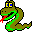#Boost C++ Libraries

...one of the most highly regarded and expertly designed C++ library projects in the world.#prim_minimum_spanning_tree

```// named parameter version
template <class Graph, class PredMap, class P, class T, class R>
void prim_minimum_spanning_tree(const Graph& g, PredMap p_map,
const bgl_named_params<P, T, R>& params)

// non-named parameter version
template <class Graph, class DijkstraVisitor,
class PredecessorMap, class DistanceMap,
class WeightMap, class IndexMap>
void prim_minimum_spanning_tree(const Graph& g,
typename graph_traits<Graph>::vertex_descriptor s,
PredecessorMap predecessor, DistanceMap distance, WeightMap weight,
IndexMap index_map, DijkstraVisitor vis)
```

This is Prim's algorithm [25,8,27,15] for solving the minimum spanning tree problem for an undirected graph with weighted edges. A MST is a set of edges that connects all the vertices in the graph where the total weight of the edges in the tree is minimized. See Section Minimum Spanning Tree Problem for more details. The implementation is simply a call to dijkstra_shortest_paths() with the appropriate choice of comparison and combine functors. The pseudo-code for Prim's algorithm is listed below. The algorithm as implemented in Boost.Graph does not produce correct results on graphs with parallel edges.

 ```PRIM-MST(G, s, w) for each vertex u in V[G] color[u] := WHITE d[u] := infinity color[s] := GRAY d[s] := 0 ENQUEUE(PQ, s) p[s] := s while (PQ != Ø) u := DEQUEUE(PQ) for each v in Adj[u] if (w(u,v) < d[v]) d[v] := w(u,v) p[v] := u if (color[v] = WHITE) ENQUEUE(PQ, v) color[v] := GRAY else if (color[v] = GRAY) UPDATE(PQ, v) else do nothing end for color[u] := BLACK end while return (p, d) ``` ``` initialize vertex u start vertex s discover vertex s examine vertex u examining edge (u,v) edge (u,v) relaxed discover vertex v edge (u,v) not relaxed finish u ```

### Parameters

IN: const Graph& g
An undirected graph. The type Graph must be a model of Vertex List Graph and Incidence Graph. It should not contain parallel edges.
Python: The parameter is named graph.
OUT: PredecessorMap p_map
The predecessor map records the edges in the minimum spanning tree. Upon completion of the algorithm, the edges (p[u],u) for all u in V are in the minimum spanning tree. If p[u] = u then u is either the root of the tree or is a vertex that is not reachable from the root. The PredecessorMap type must be a Read/Write Property Map with key and vertex types the same as the vertex descriptor type of the graph.
Python: Must be a vertex_vertex_map for the graph.

### Named Parameters

IN: root_vertex(vertex_descriptor r)
The vertex that will be the root of the minimum spanning tree. The choice of the root vertex is arbitrary.
Default: *vertices(g).first
IN: weight_map(WeightMap w_map)
The weight or ``length'' of each edge in the graph. The type WeightMap must be a model of Readable Property Map. The edge descriptor type of the graph needs to be usable as the key type for the weight map. The value type for the map must be Addable with the value type of the distance map.
Default: get(edge_weight, g)
Python: Must be an edge_double_map for the graph.
Python default: graph.get_edge_double_map("weight")
IN: vertex_index_map(VertexIndexMap i_map)
This maps each vertex to an integer in the range [0, num_vertices(g)). This is necessary for efficient updates of the heap data structure when an edge is relaxed. The type VertexIndexMap must be a model of Readable Property Map. The value type of the map must be an integer type. The vertex descriptor type of the graph needs to be usable as the key type of the map.
Default: get(vertex_index, g) Note: if you use this default, make sure your graph has an internal vertex_index property. For example, adjacenty_list with VertexList=listS does not have an internal vertex_index property.
Python: Unsupported parameter.
UTIL/OUT: distance_map(DistanceMap d_map)
The weight of the spanning tree edge into each vertex in the graph g is recorded in this property map, with edges directed away from the spanning tree root. The type DistanceMap must be a model of Read/Write Property Map. The vertex descriptor type of the graph needs to be usable as the key type of the distance map. The value type of the distance map must be Less Than Comparable.
Default: iterator_property_map created from a std::vector of the WeightMap's value type of size num_vertices(g) and using the i_map for the index map.
Python: Must be a vertex_double_map for the graph.
UTIL/OUT: color_map(ColorMap c_map)
This is used during the execution of the algorithm to mark the vertices. The vertices start out white and become gray when they are inserted in the queue. They then turn black when they are removed from the queue. At the end of the algorithm, vertices reachable from the source vertex will have been colored black. All other vertices will still be white. The type ColorMap must be a model of Read/Write Property Map. A vertex descriptor must be usable as the key type of the map, and the value type of the map must be a model of Color Value.
Default: an iterator_property_map created from a std::vector of default_color_type of size num_vertices(g) and using the i_map for the index map.
Python: The color map must be a vertex_color_map for the graph.
OUT: visitor(DijkstraVisitor v)
Use this to specify actions that you would like to happen during certain event points within the algorithm. The type DijkstraVisitor must be a model of the Dijkstra Visitor concept. The visitor object is passed by value .
Default: dijkstra_visitor<null_visitor>
Python: The parameter should be an object that derives from the DijkstraVisitor type of the graph.

### Complexity

The time complexity is O(E log V).

### Example

The file examples/prim-example.cpp contains an example of using Prim's algorithm.

### Notes

 Since the visitor parameter is passed by value, if your visitor contains state then any changes to the state during the algorithm will be made to a copy of the visitor object, not the visitor object passed in. Therefore you may want the visitor to hold this state by pointer or reference.